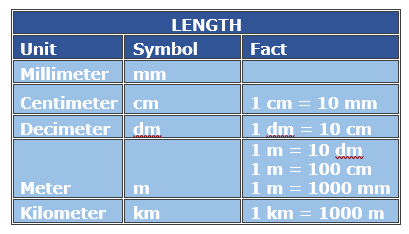# Metric Distance Conversion with Decimal Values

#### Complete Python Prime Pack

9 Courses     2 eBooks

#### Artificial Intelligence & Machine Learning Prime Pack

6 Courses     1 eBooks

#### Java Prime Pack

9 Courses     2 eBooks

The basic metric units for length are millimeters, centimeters, meters and kilometers. These units are related to each other by a factor of ten. For example, a centimeter is ten times of a millimeter and one-hundredth of a meter.

In this lesson, we solve problems involving distance conversions with decimal values.Fill in the blank to make the conversion true.

39 centimeters = ____meters

### Solution

Step 1:

100 cm = 1 meter; 1 cm = 1/100 m

Step 2:

39 centimeters = 39 × 1/100 m = 0.39 m

So, 39 cm = 0.39 m

Fill in the blank to make the conversion true.

48 millimeters = ____centimeters

### Solution

Step 1:

10 millimeter = 1 cm; 1 mm = 1/10 cm

Step 2:

48 mm = 48 mm × 1/10 = 4.8 cm

So, 48 mm = 4.8 cm

Fill in the blank to make the conversion true.

184 centimeter = ____meters

### Solution

Step 1:

100 centimeter = 1 meter; 1 cm = 1/100 m

Step 2:

184 cm = 184 × 1/100 = 1.84 m

So, 184 cm = 1.84 m